Win up to 100% scholarship on Aakash BYJU'S JEE/NEET courses with ABNAT Win up to 100% scholarship on Aakash BYJU'S JEE/NEET courses with ABNAT

# JEE Advanced Previous Year Questions with Solutions on Alternating Current

An alternating current can be defined as a current that changes its magnitude and polarity at regular intervals of time. It can also be defined as an electrical current that repeatedly changes or reverses its direction opposite to that of a Direct Current. Alternating current can be produced or generated by using devices that are known as alternators. AC is the form of current that are mostly used in different appliances.

Question 1) The inductance of two LR circuits are placed next to each other, as shown in the figure. The value of the self-inductance of the inductors, resistance, mutual-inductance and applied voltages are specified in the given circuit. After both the switches are closed simultaneously the total work done by the batteries against the induced EMF in the inductors by the time the currents reach their steady-state values is ______ mJ.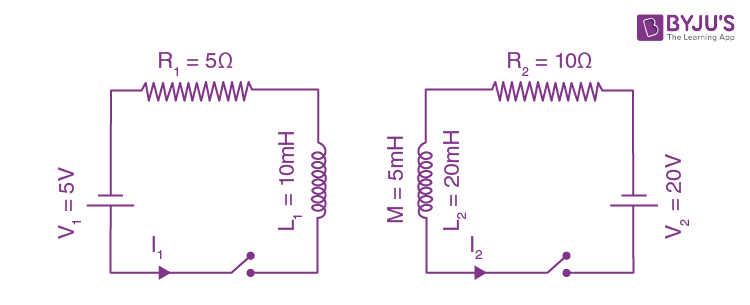Solution:

Given,

Mutual Inductance, M = 5mH

L1 = 10 mH

V1 = 5 V

L2 = 20 mH

V2 = 20 V

I1 = V1/R1 = 5/5 = 1A

I2= V2/R2 = 20/10 = 2A

After both the switches are closed simultaneously, the total work done by the batteries against the induced EMF = Increase in the magnetic energy

Therefore, W = ΔU = (½) L1I12 + (½) L2I22 + MI1I2

= (½)(10 x 10-3)12 + (½)(20 x 10-3)(22) + (5 x 10-3) x 1 x 2

= (5 + 40 + 10) x 10-3

= 55 mJ

Question 2) A 20 Henry inductor coil is connected to a 10 ohm resistance in series as shown in the figure. The time at which rate of dissipation of energy (Joule’s heat) across the resistance is equal to the rate at which magnetic energy is stored in the inductor, is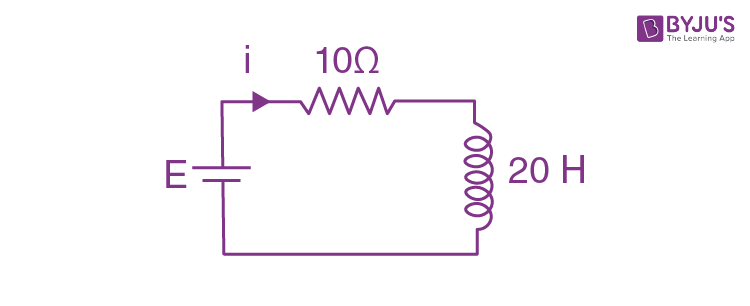(A) 2/ln 2

(B) (½) ln2

(C) 2ln 2

(D) ln 2

Solution:

i = i0 (1 – e-t/τ)

di/dt = (i0/τ) e-t/τ

di/dt = [E/(10 x 2)] e-t/2 ——–(1) [since τ = L/R = 20/10 = 2] [L(di/dt)i] = i2R

⇒ L(di/dt) = iR ———(2)

From equation (1) and (2) we get

L [E/(10 x 2)] e-t/2 = iR

(L/R)[E/(20)] e-t/2 = i0 (1 – e-t/τ) [since τ = L/R = 20/10 = 2] [E/10] e-t/2 = [E/10) (1 – e-t/τ)

e-t/2 = ½

t/2 = ln 2

Question 3) In the figure shown, a circuit contains two identical resistors with resistance R = 5 ohm and inductance with L = 2 mH. An ideal battery of 15 V is connected in the circuit. What will be the current through the battery long after the switch is closed?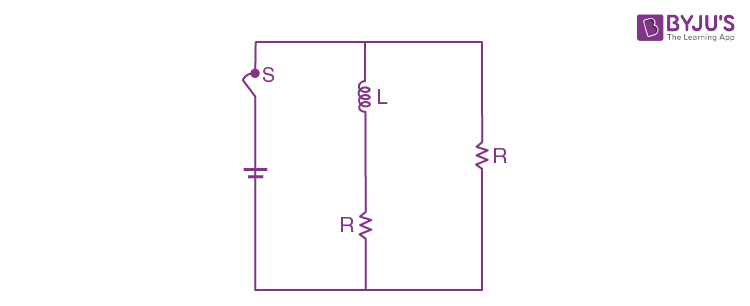(A) 5.5 A

(B) 7.5 A

(C) 3 A

(D) 6 A

Solution:

For a long time after the switch is closed, the inductor will be idle so the equivalent diagram will be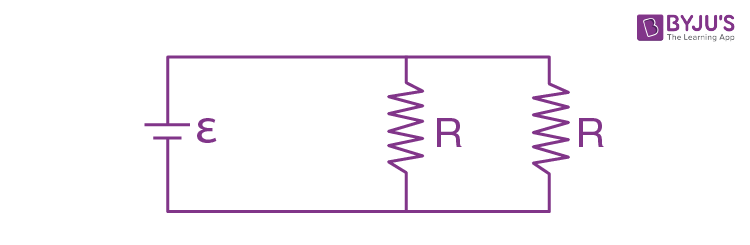I = ε/[(R x R)/(R + R)]

= 2ε/R

= (2 x 15)/5

= 6 A

Question 4)An AC voltage source of variable angular frequency and fixed amplitude V0 is connected in series with a capacitance C and an electric bulb of resistance R (inductance zero). When ω is increased

(A) the bulb glows dimmer

(B) the bulb glows brighter

(C) total impedance of the circuit is unchanged

(D) total impedance of the circuit increases

Answer: (B) the bulb glows brighter

Solution:

Impedance

$$\begin{array}{l}Z = \sqrt{\frac{1}{(\omega C)^{2}}+R^{2}}\end{array}$$

As ω increases, Z decreases

Hence, the bulb will glow brighter

Question 5) A series R-C combination is connected to an AC voltage of angular frequency ω=500 radian/s. If the impedance of the R-C circuit is R√1.25 the time constant (in millisecond) of the circuit is?

(A) 1

(B) 2

(C) 3

(D) 4

Solution:

The capacitance of the capacitor is C

Xc = 1/ωC = 1/500C

Impedance of the circuit, Z = R√1.25

Using Z2 = R2 + Xc2

1.25R2 = R2 + 1/(500)2C2

0.25R2 = 1/(0.25 x 106)C2

R2C2 = 10-6/(0.25)2

⇒ RC = 10-3/0.25

= 0.004 s

= 4 ms

Question 6) In the given circuit, the AC source has ω = 100 rad/s. Considering the inductor and capacitor to be ideal, the correct choice (s) is(are)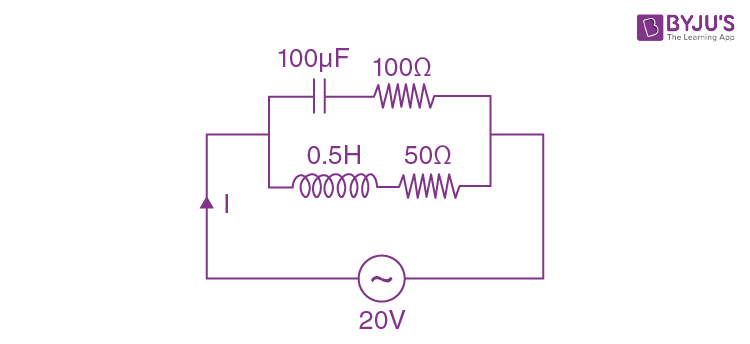(A) The current through the circuit, I is 0.3 A.

(B) The current through the circuit, is 0.3 √2A

(C) The voltage across 100Ω resistor 10√2 V

(D) The voltage across 50Ω resistor 10 V

Solution:

In the upper branch the net impedance, (C = 100μF, R = 100Ω)

Therefore, the net impedance will be

$$\begin{array}{l}Z_{1} = \sqrt{\frac{1}{(\omega C)^{2}}+R^{2}}\end{array}$$
$$\begin{array}{l}Z_{1} = \sqrt{\frac{1}{(100\times 100\times 10^{-6})^{2}}+100^{2}}\end{array}$$

= 100√2

Current I1 = V/Z1 = 20/(100√2)

cos Φ1 = R/(100√2)

= 100/(100√2)

= 1/√2

⇒Φ1 = 450

In the lower branch the net impedance (L = 0.5 H, R = 50 Ω)

Therefore, the net impedance will be

$$\begin{array}{l}Z_{2} = \sqrt{(\omega L)^{2}+R^{2}}\end{array}$$
$$\begin{array}{l}Z_{2} = \sqrt{(0.5\times 100)^{2}+100^{2}}\end{array}$$

= 50√2

Current, I2 = V/Z2

= 20/(50√2)

cos Φ1 = R/(50√2)

= 50/(50√2)

= 1/√2

⇒Φ1 = 450

Thus the total current I is given by the summation of I1 and I2 which differ by 900 in phase and hence

$$\begin{array}{l}I = \sqrt{I_{1}^{2}+I_{2}^{2}}\end{array}$$

= (1/√10) A ≈ 0.3 A

Voltage across 100 Ω = I1R1 = [20/(100√2)] x 100 = 10√2 V

Voltage across 50 Ω = I2R2 = [20/(50√2)] x 50 = 10√2 V

Question 7) An alternating voltage v(t) = 220 sin 100 πt volt is applied to a purely resistive load of 50 Ω. The time taken for the current to rise from half of the peak value to the peak value is

(A) 5 ms

(B) 2.2 ms

(C) 7.2 ms

(D) 3.3 ms

Solution:

Given,

V(t) = 220 sin 100πt

I(t) = (220/50) sin 100πt

Phase to be covered θ = 600 = π/3

Time taken, t = θ/ω

= (π/3)/100π

= (1/300) sec

= 3.3 ms

Question 8) A sinusoidal voltage V(t) = 100 sin (500t) is applied across a pure inductance of L= 0.02 H. The current through the coil is

(A) 10 cos (500 t)

(B) -10 cos (500 t)

(C) 10 sin (500 t)

(D) – 10 sin (500 t)

Answer: (B) -10 cos (500 t)

Solution:

In a pure inductive circuit current always lags behind the emf by π/2

If v(t) = v0sinωt

Then, I(t) = I0sin(ωt – π/2)

Given, V(t) = 100 sin (500t)

And I0 = E0/ωL

= 100/ (500 x 0.02) = 10sin(500t – π/2)

I0 =- 10 cos (500 t)

Question 9) An AC circuit has R = 100 Ω, C= 2μF and L= 80 mH, connected in series. The quality factor of the circuit is

(A) 2

(B) 0.5

(C) 20

(D) 400

Solution:

Quality factor,

$$\begin{array}{l}Q = \frac{1}{R}\sqrt{\frac{L}{C}}\end{array}$$

$$\begin{array}{l}Q = \frac{1}{100}\sqrt{\frac{80\times 10^{-3}}{2\times 10^{-6}}}\end{array}$$

$$\begin{array}{l}Q = \frac{1}{100}\sqrt{40\times 10^{3}}\end{array}$$

= 200/100 = 2

Question 10) When the rms voltage VL, VC and VR are measured respectively across the inductor L, the capacitor C and the resistor R in a series LCR circuit connected to an AC source, it is found that the ratio VL: VC: VR = 1: 2: 3. If the rms voltage of the AC sources is 100 V, The VR is close to

(A) 50 V

(B) 70 V

(C) 90 V

(D) 100 V

Solution:

Given,

VL: VC: VR = 1: 2: 3

V = 100 V

⇒ VR = 3K, VL = K, VC = 2K

We know,

$$\begin{array}{l}V = \sqrt{V_{R}^{2}+(V_{L}-V_{C})^{2}}\end{array}$$
$$\begin{array}{l}100 = \sqrt{9K^{2}+K^{2}}\end{array}$$

100 = √10 K

K =100/√10

VR = 3K = (3 x 100)/√10

= 94.86 volts

So, VR is close to 90 V